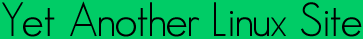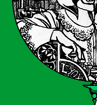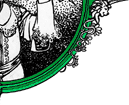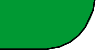Quick ?s
Cheat Sheets
Man Pages
The Lynx
Software
 ```REMAINDER(3) Linux Programmers Manual REMAINDER(3) NAME drem, dremf, dreml, remainder, remainderf, remainderl - floating-point remainder function SYNOPSIS #include /* The C99 versions */ double remainder(double x, double y); float remainderf(float x, float y); long double remainderl(long double x, long double y); /* Obsolete synonyms */ double drem(double x, double y); float dremf(float x, float y); long double dreml(long double x, long double y); Link with -lm. Feature Test Macro Requirements for glibc (see feature_test_macros(7)): remainder(), remainderf(), remainderl(): _SVID_SOURCE || _BSD_SOURCE || _XOPEN_SOURCE >= 500 || _ISOC99_SOURCE; or cc -std=c99 drem(), dremf(), dreml(): _SVID_SOURCE || _BSD_SOURCE DESCRIPTION The remainder() function computes the remainder of dividing x by y. The return value is x - n * y, where n is the value x / y, rounded to the nearest integer. If this quotient is 1/2 (mod 1), it is rounded to the nearest even number (independent of the current rounding mode). If the return value is 0, it has the sign of x. The drem() function does precisely the same thing. RETURN VALUE The remainder() function returns the remainder, unless y is zero, when the function fails and errno is set. ERRORS EDOM The denominator y is zero. CONFORMING TO IEC 60559. The functions remainder(), remainderf(), and remainderl() are from C99. The function drem() is from 4.3BSD. The float and long double variants dremf() and dreml() exist on some systems, such as Tru64 and glibc2. Avoid the use of these functions in favor of remainder() etc. EXAMPLE The call "remainder(29.0, 3.0)" returns -1. SEE ALSO fmod(3), remquo(3) COLOPHON This page is part of release 3.05 of the Linux man-pages project. A description of the project, and information about reporting bugs, can be found at http://www.kernel.org/doc/man-pages/. 2008-06-16 REMAINDER(3) ```Yals.net is © 1999-2009 Crescendo Communications Sharing tech info on the web for more than a decade! This page was generated Thu Apr 30 17:05:25 2009